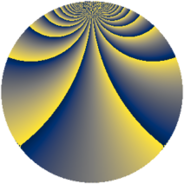# Properties

 Label 2736.3.drLevel $2736$ Weight $3$ Character orbit 2736.dr Rep. character $\chi_{2736}(227,\cdot)$ Character field $\Q(\zeta_{12})$ Dimension $3824$ Sturm bound $1440$

# Related objects

## Defining parameters

 Level: $$N$$ $$=$$ $$2736 = 2^{4} \cdot 3^{2} \cdot 19$$ Weight: $$k$$ $$=$$ $$3$$ Character orbit: $$[\chi]$$ $$=$$ 2736.dr (of order $$12$$ and degree $$4$$) Character conductor: $$\operatorname{cond}(\chi)$$ $$=$$ $$2736$$ Character field: $$\Q(\zeta_{12})$$ Sturm bound: $$1440$$

## Dimensions

The following table gives the dimensions of various subspaces of $$M_{3}(2736, [\chi])$$.

Total New Old
Modular forms 3856 3856 0
Cusp forms 3824 3824 0
Eisenstein series 32 32 0

## Trace form

 $$3824q - 4q^{4} - 12q^{5} - 8q^{6} - 8q^{7} + O(q^{10})$$ $$3824q - 4q^{4} - 12q^{5} - 8q^{6} - 8q^{7} - 12q^{11} - 4q^{16} - 8q^{19} - 264q^{20} - 24q^{23} + 8q^{24} + 112q^{28} + 172q^{30} - 72q^{36} - 6q^{38} - 16q^{39} + 336q^{42} - 4q^{43} + 92q^{45} - 13056q^{49} - 316q^{54} - 32q^{55} - 4q^{58} - 4q^{61} - 16q^{64} + 864q^{66} + 180q^{68} - 516q^{74} + 154q^{76} - 12q^{77} - 16q^{81} - 48q^{82} - 12q^{83} + 96q^{85} - 912q^{87} - 12q^{92} + 28q^{93} - 812q^{96} + 188q^{99} + O(q^{100})$$

## Decomposition of $$S_{3}^{\mathrm{new}}(2736, [\chi])$$ into newform subspaces

The newforms in this space have not yet been added to the LMFDB.